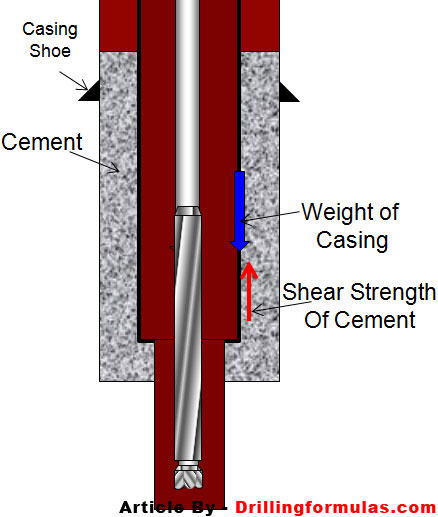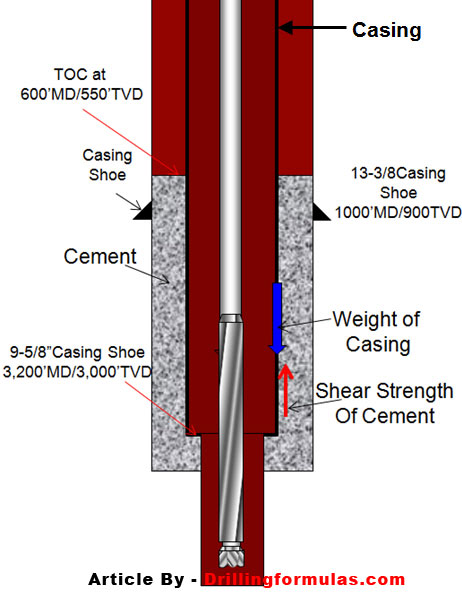# Minimum strength to support casing string

One function of cement is to support casing string and the shear strength of cement holds casing string once cement is set. You may think about other load supporting casing as compressive loading at coupling areas or thermal movement. They are the part of the supporting force as well but the most support is from the cement shear strength.(Diagram above shows how shear strength of cement supports casing string)

We don’t normally measure shear strength of cement but we can apply knowledge from Civil engineer to estimate the shear strength from the compressive strength. Generally, cement has the shear strength approximately 1/12 of compressive strength. For instant, if the cement 1000 psi compressive strength, its shear strength is 83.3 (1000 x 1/12) psi.

Let’s take a look into the detailed calculation for more understanding.

The information given is listed below

Casing 9-5/8”, 40 ppf (pound per foot), ID of casing = 8.835”

Casing is set at 3,200’MD/3,000’TVD

Top of cement at 600’MD/550’TVD

Previous casing shoe (13-3/8”) = 1000’MD/900TVD(The diagram shows all figures as per the data)

We will use the pressure and force concept (Force = Pressure x Area).

1. The outer surface area of casing covered with cement is equal to circumference of casing multiplied by length of cement covering casing.

Area = Π x D x L

Where

Area in square inch.

Π (called pi) =22/7

D is diameter in inch.

L is length of cement in inch

Area = Π x 9.625 x (2600 x 12)

Area = 943,420 square inch.

2. Casing weight = weight in ppf x total length of casing

Casing weight = 40 x 3200 = 128,000 lb

3. Shear force is required to support whole weight of casing

Shear strength (lb/square inch) = Casing weight ÷ Area of casing covered by cement

Shear strength (psi) = 128,000 ÷ 943,420 = 0.136 psi

We can estimate the compressive strength of cement based on 1/12 figure.

Shear Strength ÷ Compressive Strength =1/12

Compressive strength = 12 x 0.136 = 1.63 psi

The figure tells us that you need only 1.63 psi compressive strength to support whole casing string.

Ps, This is rough estimation without any consideration regarding complex load, thermal movement, etc.

Ref books:Cementing Technology Books

Share the joyWorking in the oil field and loving to share knowledge.

### 5 Responses to Minimum strength to support casing string

1.Ibrahim says:

That is very usefull thanks

2.ppa says:

Great….i like it

3.ppa says:

Great….i like it so much……

4.fathy Gamal says:

many thanks for all these valuable calculations and informations

5.Yapon says:

Thank you

This site uses Akismet to reduce spam. Learn how your comment data is processed.